Next: Is there any efficient Up: Convex Polyhedron Previous: Is there any efficient   Contents

## Is there any efficient algorithm to compute the intersection of two (or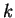) polytopes

Let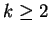, and let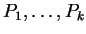be input polytopes in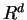, and let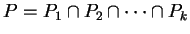be the polytope we want to compute.

This problem of computing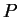needs to be specified further. Namely, what is the representation of input and that of output?

If the input polytopes are H-polytopes (given by inequalities) then the intersection is represented by the union of the two inequality systems. To get a minimal H-reprentation for the intersection is just a redundancy removal given in Section 2.21. To get a minimal V-representation for the intersection is the vertex enumeration problem explained in Section 2.12.

An interesting case is when both input and output polytopes are V-polytopes (i.e. given by vertices and perhaps some redundant points). One primitive way to solve this problem consists of two steps: (1) generate minimal H-representations of each of the inputpolytopes, (2) solve the vertex enumeration problem for the union of theH-representations. This naive approach might be satisfactory for small dimensions or not-too-complicated polytopes. Recently, a polynomial algorithm has been found for the special case when the input polyopes are in general position [FLL00]. This algorithm is not yet practical because the general position assumption does not seem to be easily simulated for the general case. It should be remarked that the dual version of this problem is to compute a minimal H-representation of the convex hull ofH-polytopes. Actually the paper [FLL00] treats this dual problem.Next: Is there any efficient Up: Convex Polyhedron Previous: Is there any efficient   Contents
Komei Fukuda 2004-08-26